# RD Sharma Solutions for Class 9 Maths Chapter 16 Circles Exercise 16.2

Get free RD Sharma Solutions for Class 9 Maths Chapter 16 exercise 16.2 – Circles here. In this exercise students will study about congruence of circles and arcs. Solving the RD Sharma class 9 Maths questions helps to build the basics and in-depth understanding of basic of circle. Click on the link given below to get your pdf now.

## Download PDF of RD Sharma Solutions for Class 9 Maths Chapter 16 Circles Exercise 16.2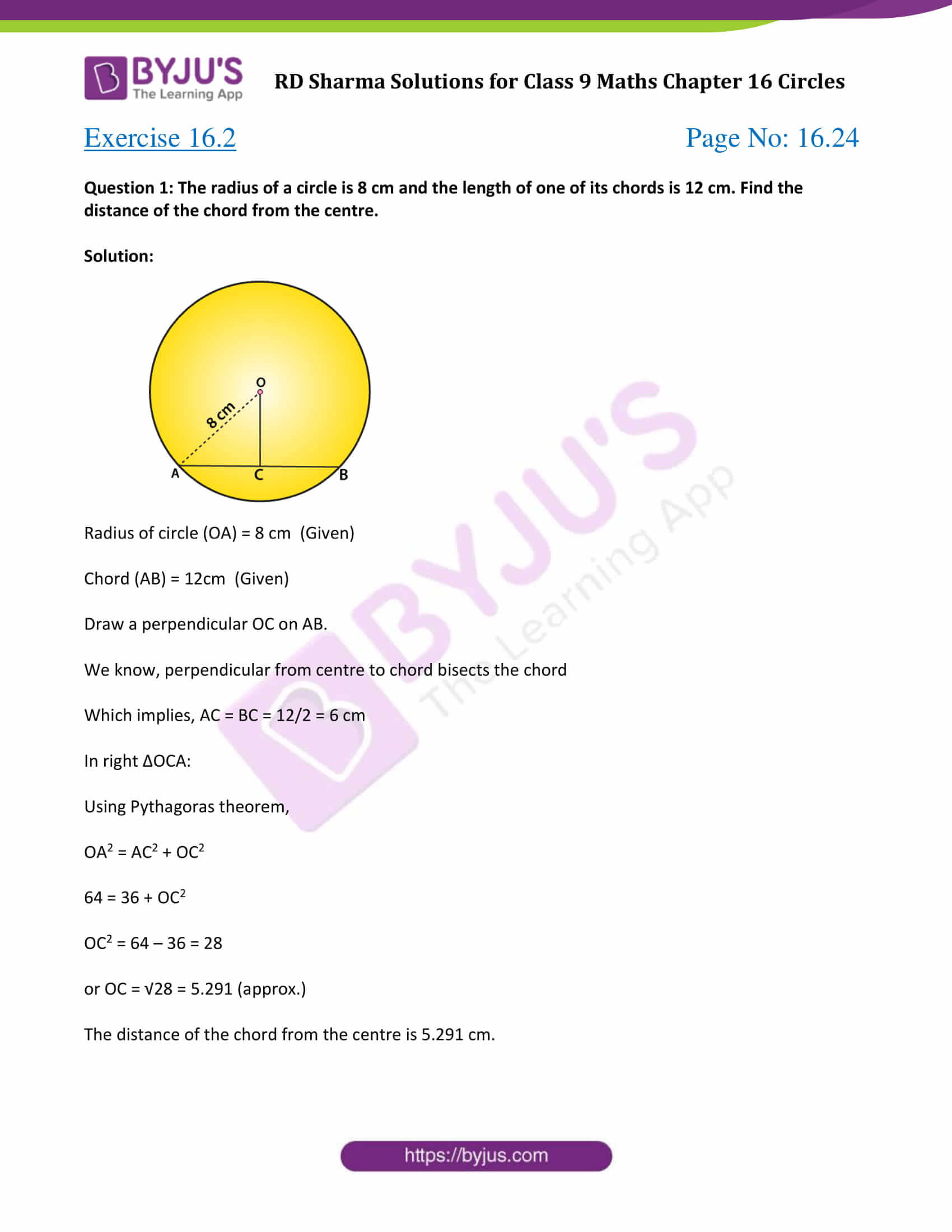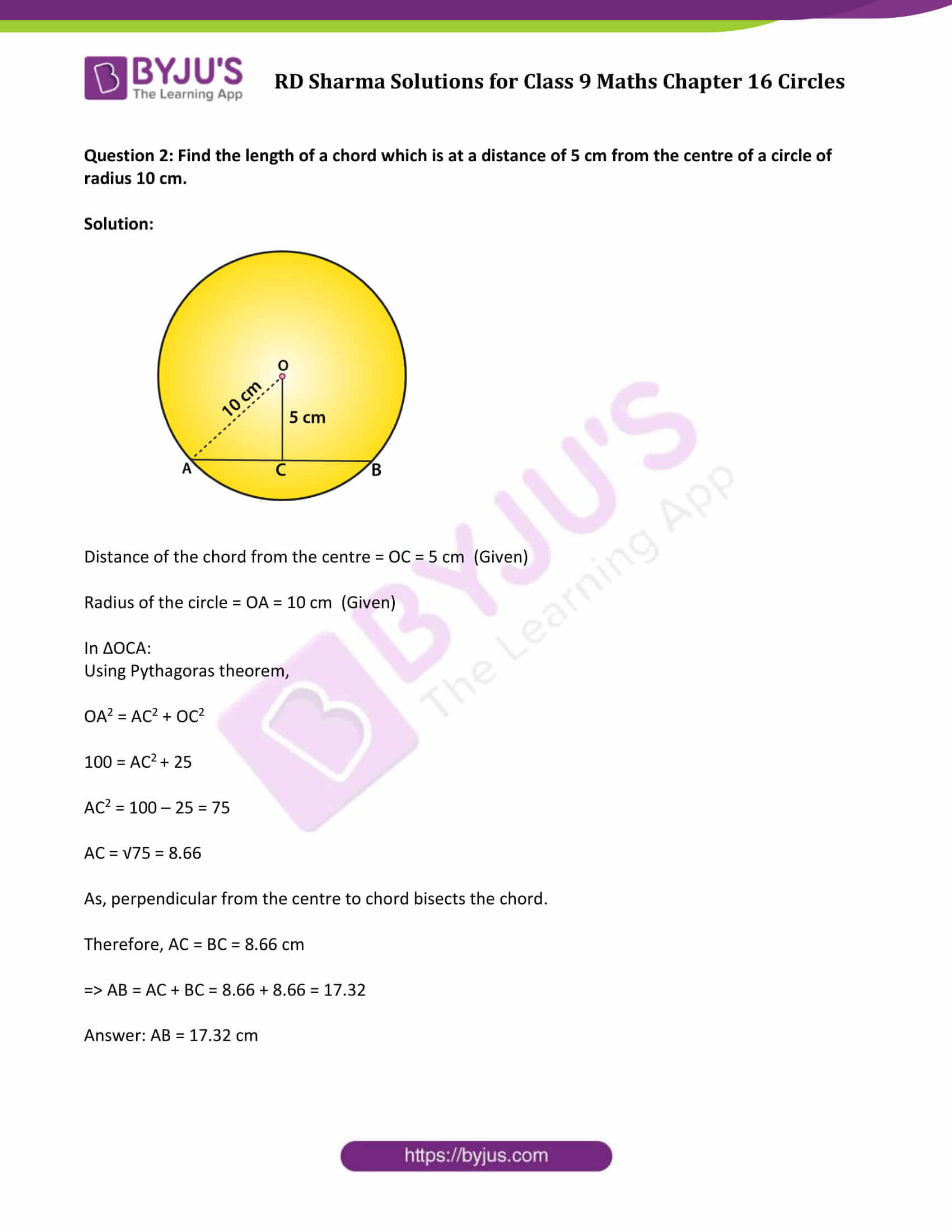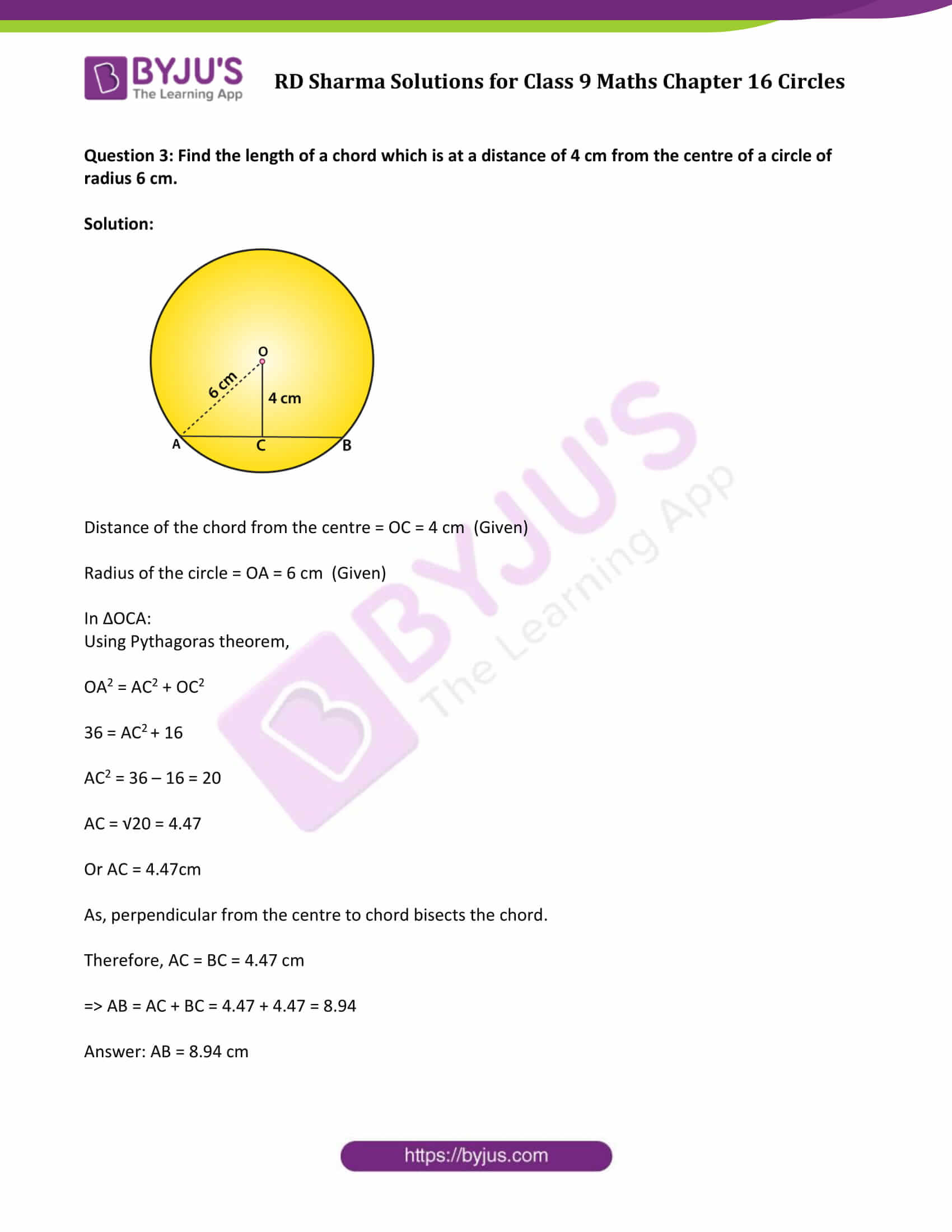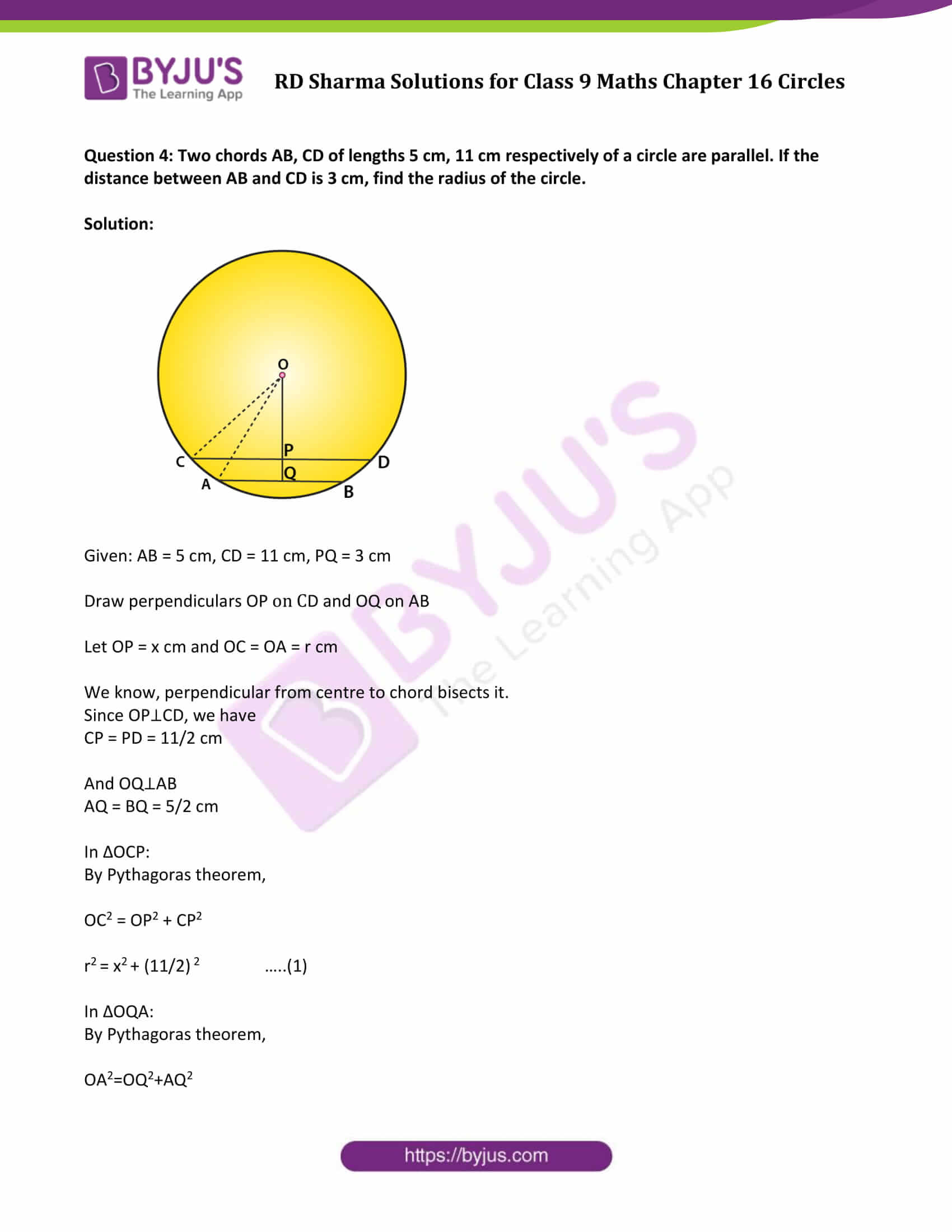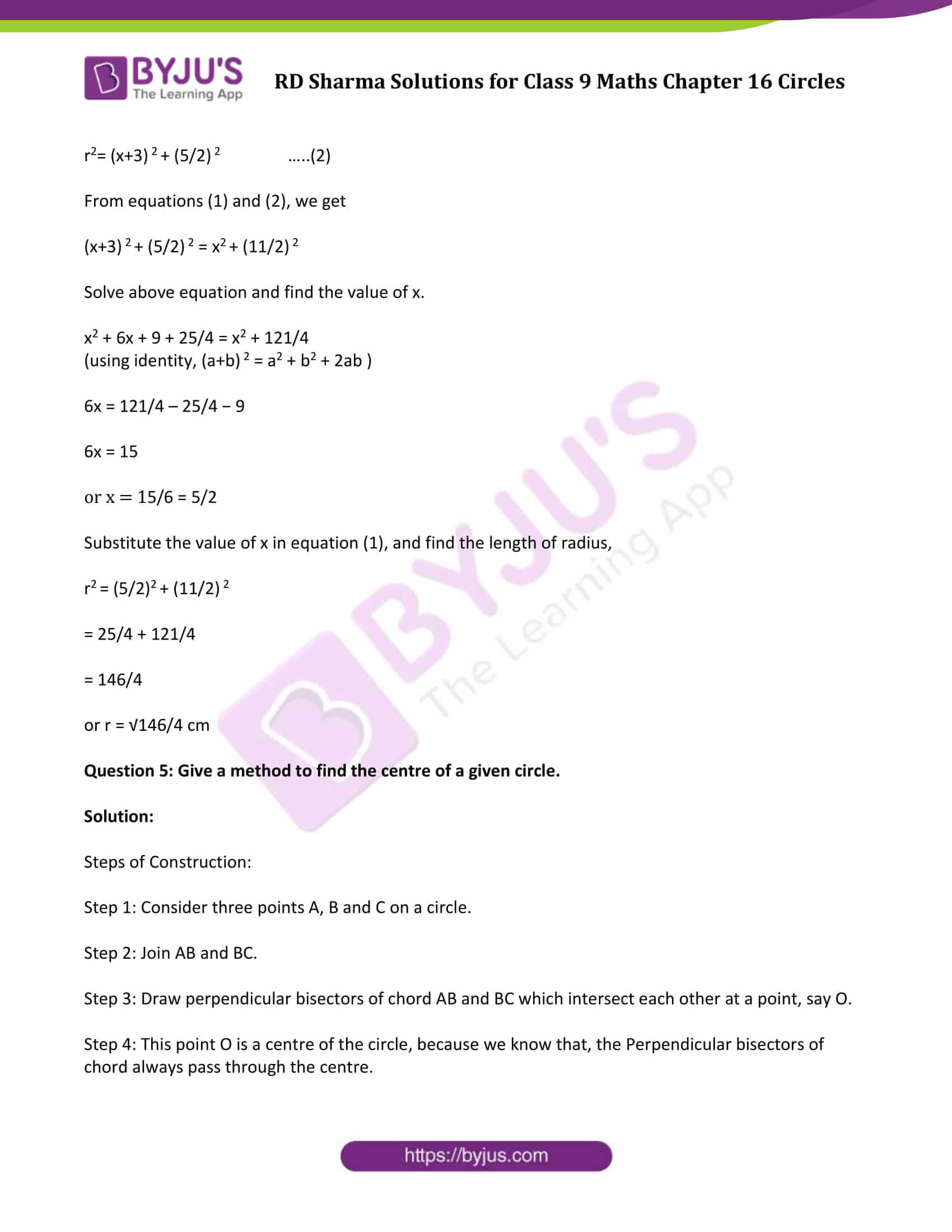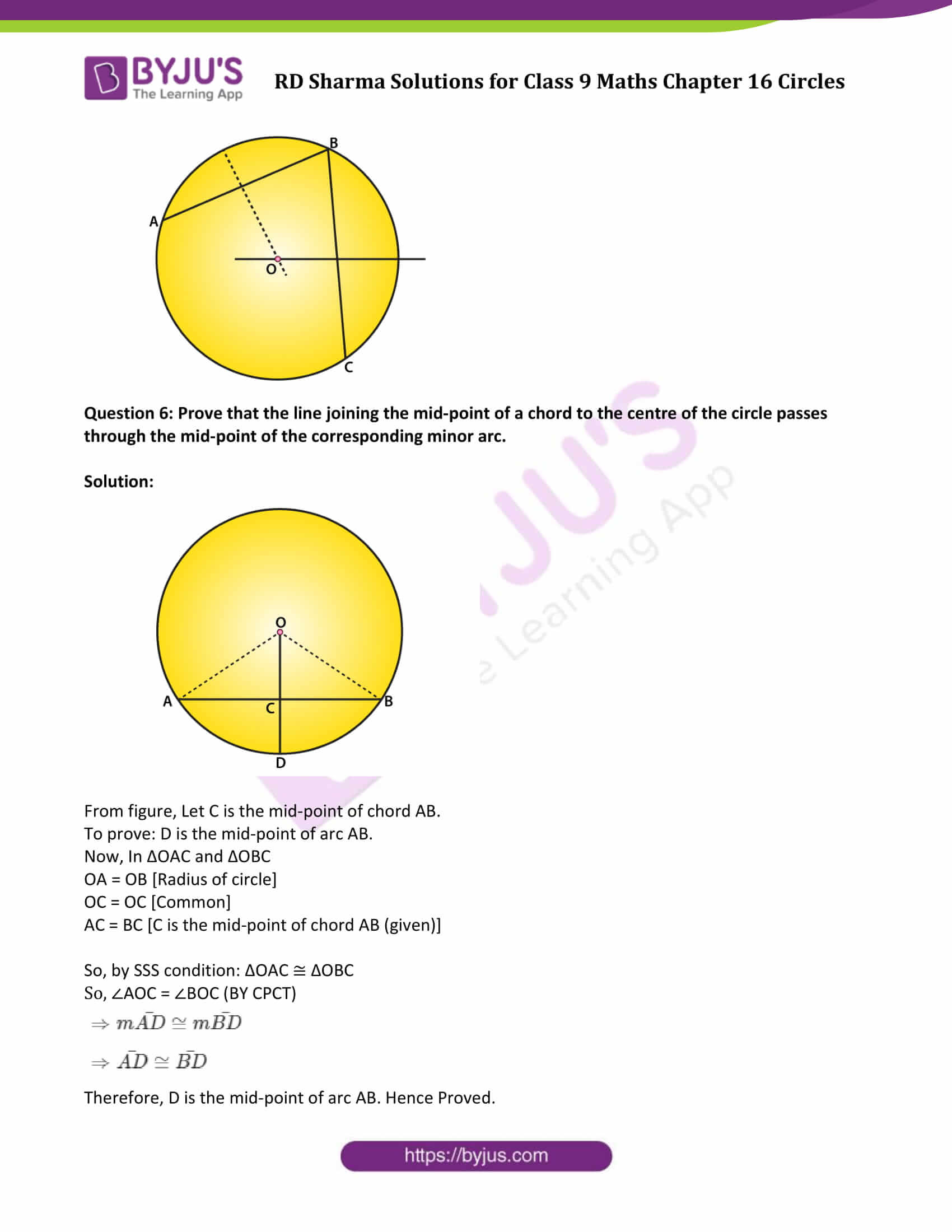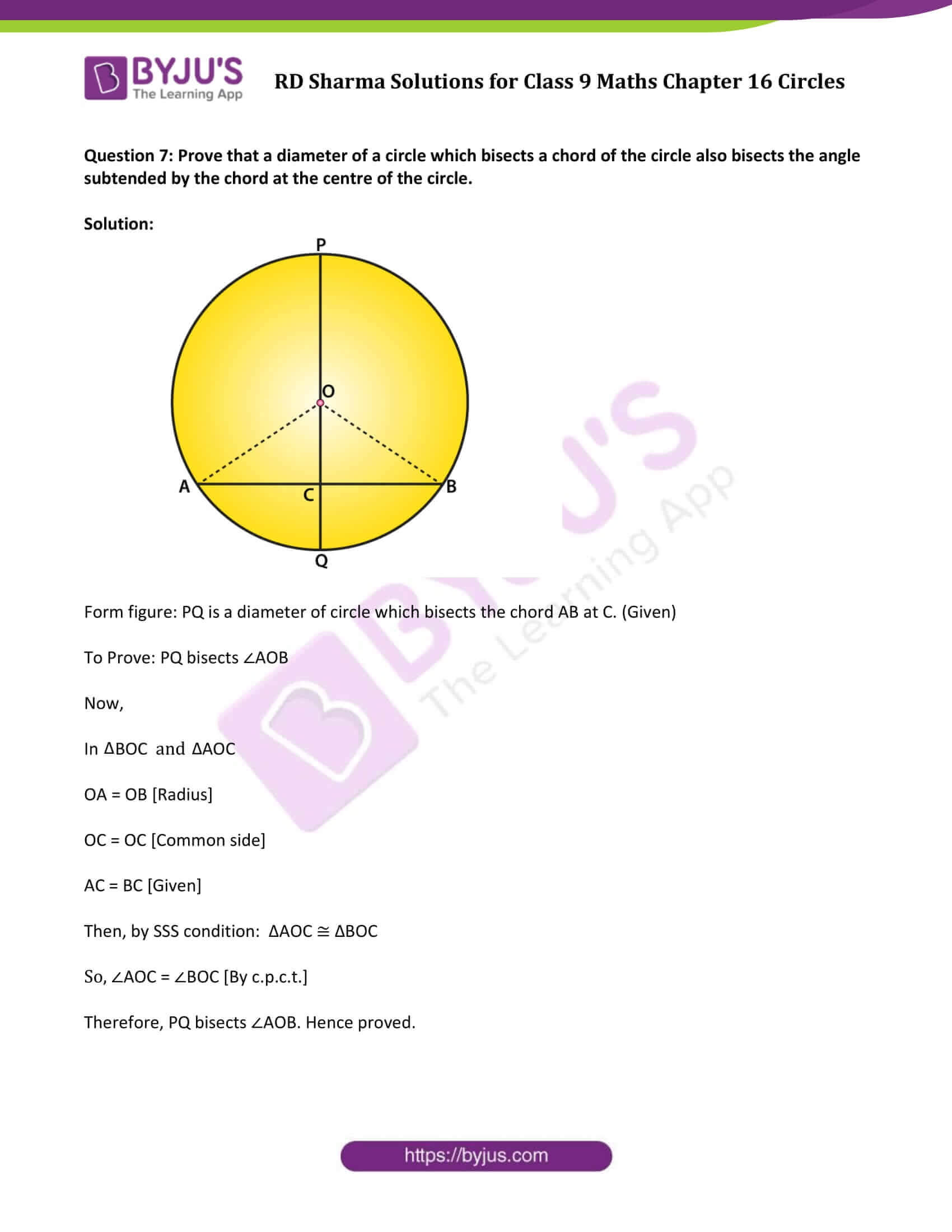### Access Answers to Maths RD Sharma Solutions for Class 9 Chapter 16 Circles Exercise 16.2 Page number 16.24

Question 1: The radius of a circle is 8 cm and the length of one of its chords is 12 cm. Find the distance of the chord from the centre.

Solution: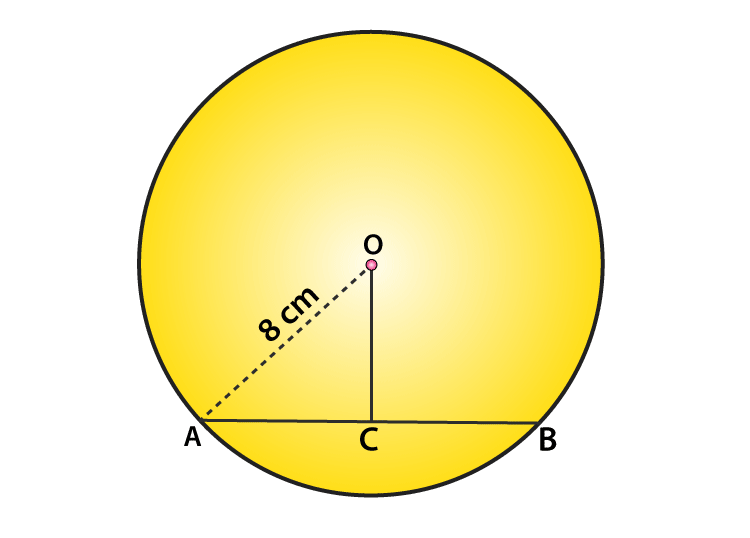Radius of circle (OA) = 8 cm (Given)

Chord (AB) = 12cm (Given)

Draw a perpendicular OC on AB.

We know, perpendicular from centre to chord bisects the chord

Which implies, AC = BC = 12/2 = 6 cm

In right ΔOCA:

Using Pythagoras theorem,

OA2 = AC2 + OC2

64 = 36 + OC2

OC2 = 64 – 36 = 28

or OC = √28 = 5.291 (approx.)

The distance of the chord from the centre is 5.291 cm.

Question 2: Find the length of a chord which is at a distance of 5 cm from the centre of a circle of radius 10 cm.

Solution: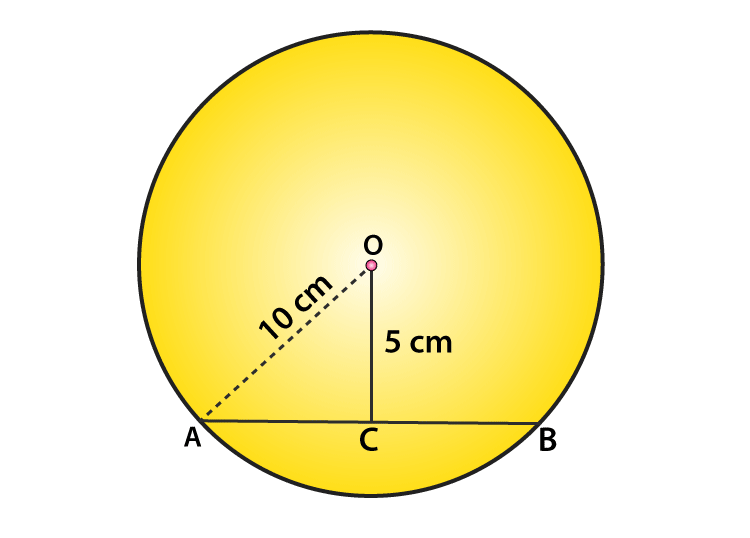Distance of the chord from the centre = OC = 5 cm (Given)

Radius of the circle = OA = 10 cm (Given)

In ΔOCA:

Using Pythagoras theorem,

OA2 = AC2 + OC2

100 = AC2 + 25

AC2 = 100 – 25 = 75

AC = √75 = 8.66

As, perpendicular from the centre to chord bisects the chord.

Therefore, AC = BC = 8.66 cm

=> AB = AC + BC = 8.66 + 8.66 = 17.32

Question 3: Find the length of a chord which is at a distance of 4 cm from the centre of a circle of radius 6 cm.

Solution: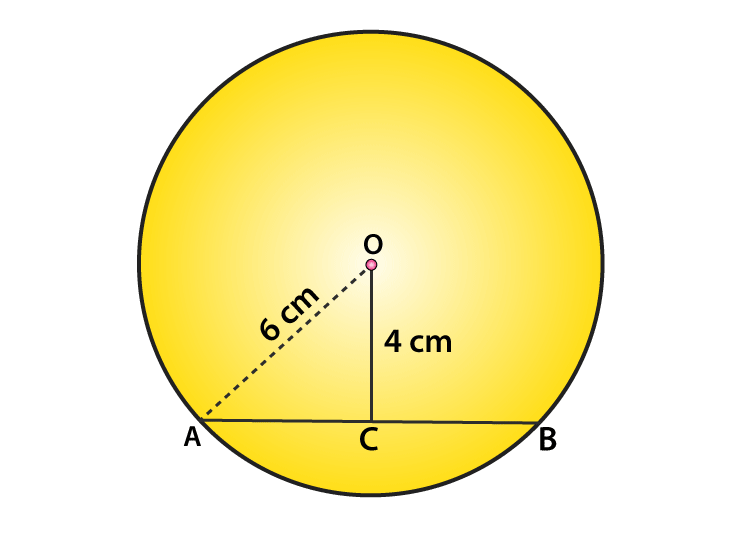Distance of the chord from the centre = OC = 4 cm (Given)

Radius of the circle = OA = 6 cm (Given)

In ΔOCA:

Using Pythagoras theorem,

OA2 = AC2 + OC2

36 = AC2 + 16

AC2 = 36 – 16 = 20

AC = √20 = 4.47

Or AC = 4.47cm

As, perpendicular from the centre to chord bisects the chord.

Therefore, AC = BC = 4.47 cm

=> AB = AC + BC = 4.47 + 4.47 = 8.94

Question 4: Two chords AB, CD of lengths 5 cm, 11 cm respectively of a circle are parallel. If the distance between AB and CD is 3 cm, find the radius of the circle.

Solution: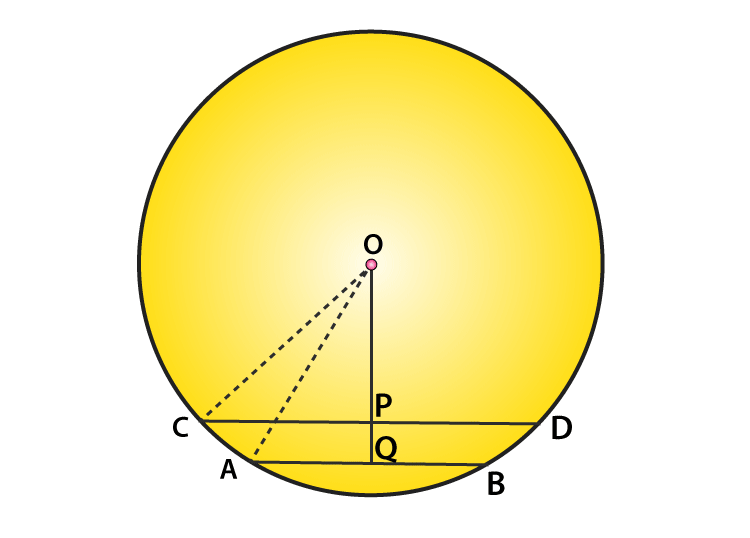Given: AB = 5 cm, CD = 11 cm, PQ = 3 cm

Draw perpendiculars OP on CD and OQ on AB

Let OP = x cm and OC = OA = r cm

We know, perpendicular from centre to chord bisects it.

Since OP⊥CD, we have

CP = PD = 11/2 cm

And OQ⊥AB

AQ = BQ = 5/2 cm

In ΔOCP:

By Pythagoras theorem,

OC2 = OP2 + CP2

r2 = x2 + (11/2) 2 …..(1)

In ΔOQA:

By Pythagoras theorem,

OA2=OQ2+AQ2

r2= (x+3) 2 + (5/2) 2 …..(2)

From equations (1) and (2), we get

(x+3) 2 + (5/2) 2 = x2 + (11/2) 2

Solve above equation and find the value of x.

x2 + 6x + 9 + 25/4 = x2 + 121/4

(using identity, (a+b) 2 = a2 + b2 + 2ab )

6x = 121/4 – 25/4 − 9

6x = 15

or x = 15/6 = 5/2

Substitute the value of x in equation (1), and find the length of radius,

r2 = (5/2)2 + (11/2) 2

= 25/4 + 121/4

= 146/4

or r = √146/4 cm

Question 5: Give a method to find the centre of a given circle.

Solution:

Steps of Construction:

Step 1: Consider three points A, B and C on a circle.

Step 2: Join AB and BC.

Step 3: Draw perpendicular bisectors of chord AB and BC which intersect each other at a point, say O.

Step 4: This point O is a centre of the circle, because we know that, the Perpendicular bisectors of chord always pass through the centre.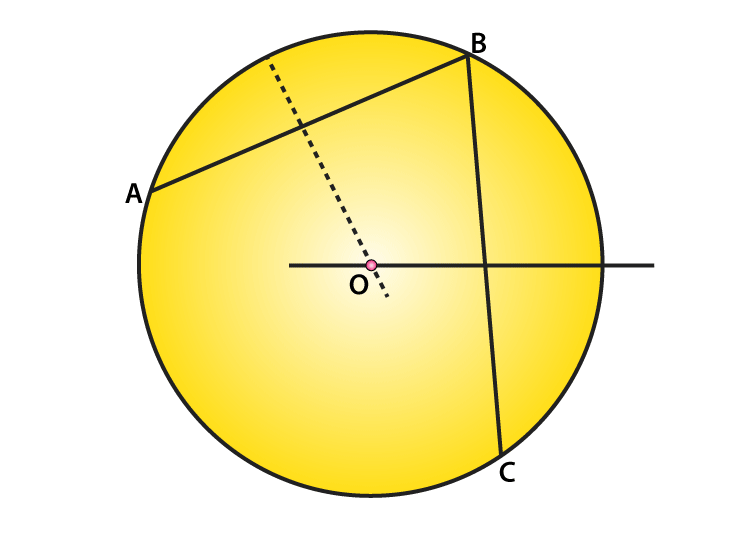Question 6: Prove that the line joining the mid-point of a chord to the centre of the circle passes through the mid-point of the corresponding minor arc.

Solution: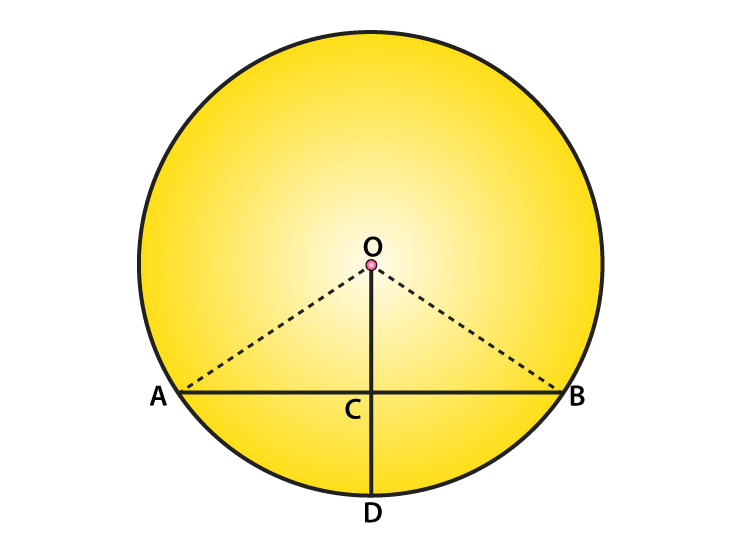From figure, Let C is the mid-point of chord AB.

To prove: D is the mid-point of arc AB.

Now, In ΔOAC and ΔOBC

OA = OB [Radius of circle]

OC = OC [Common]

AC = BC [C is the mid-point of chord AB (given)]

So, by SSS condition: ΔOAC ≅ ΔOBC

So, ∠AOC = ∠BOC (BY CPCT)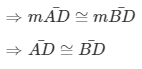Therefore, D is the mid-point of arc AB. Hence Proved.

Question 7: Prove that a diameter of a circle which bisects a chord of the circle also bisects the angle subtended by the chord at the centre of the circle.

Solution: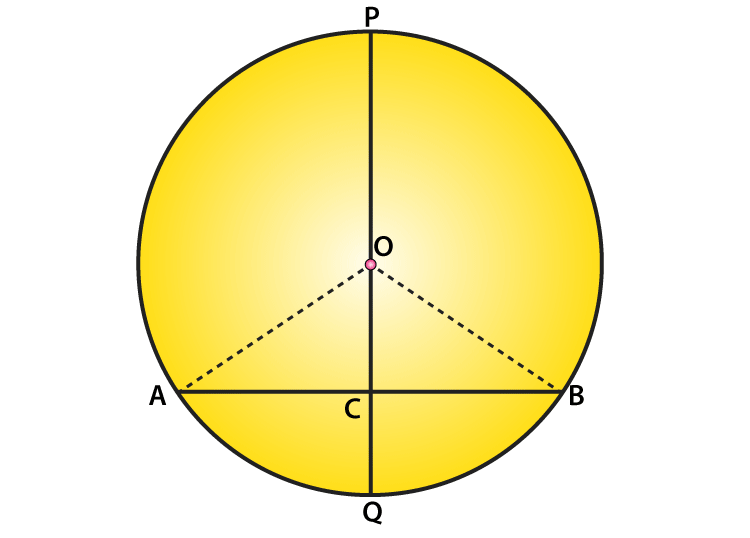Form figure: PQ is a diameter of circle which bisects the chord AB at C. (Given)

To Prove: PQ bisects ∠AOB

Now,

In ΔBOC and ΔAOC

OC = OC [Common side]

AC = BC [Given]

Then, by SSS condition: ΔAOC ≅ ΔBOC

So, ∠AOC = ∠BOC [By c.p.c.t.]

Therefore, PQ bisects ∠AOB. Hence proved.

## RD Sharma Solutions for Class 9 Maths Chapter 16 Circles Exercise 16.2

RD Sharma Solutions Class 9 Maths Chapter 16 Circles Exercise 16.2 is based on the following topics and subtopics:

• Congruence of circles and arcs

-Congruent circles

-Congruent arcs

• Some important results on Congruent arcs and chords